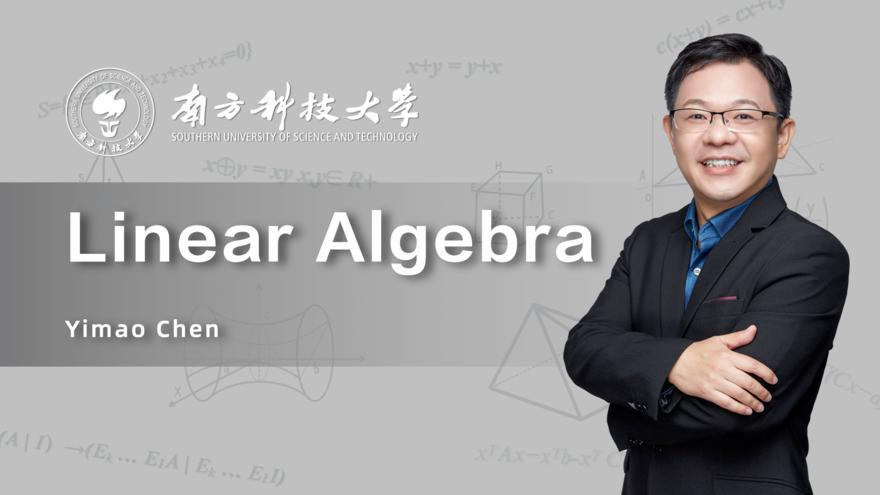Linear AlgebraLinear Algebra

 开课平台 学堂在线
 开课时间 2022/06/21 - 2022/12/31 28 周 开课中 -

To present a careful treatment of the principal topics of linear algebra and to illustrate the power of the subject through a variety of applications.  Topics including: systems of linear equations, determinants, matrices, vector spaces, linear transformations, eigenvalues and eigenvectors, and quadratic forms. Linear algebra is suitable for all students majoring in science or mathematics, or any other courses of study requiring it.

After completing this course, students will be able to understand the basic methods and techniques in linear algebra. This course also helps students develop their abilities in abstract thinking and logical reasoning.

Linear Algebra serves as a prerequisite for many courses, including numerical analysis, ordinary differential equations, partial differential equations, regression analysis, financial mathematics and financial engineering and etc.

Chapter 1 Gaussian Elimination and Matrices
01 Gaussian Elimination
02 Linear Equations
03 Vectors in R^n
04 Matrix Multiplication
05 Elementary Matrices
06 The Interplay
07 LU Factorization
08 Inverses
09 Inverses Properties
10 Example Transposes
11 Partitioned Matrices
Chapter 2 Vector Spaces
12 Vector Spaces and Examples
13 Subspaces
14 Linear Independence
15 Basis
16 Maximal Linearly Independent Subset
17 Solving Ax=0
18 Solving Ax=b
19 Four Fundamental Subspaces
Chapter 3 Orthogonality
20 Inner Product
21 Orthogonal Vectors
22 Projection Onto Lines
23 Projection Onto Column Space
24 Least-Squares
25 Orthonormal Basis
26 Gram-Schmidt Orthogonalization Procedure
27 QR Factorization
Chapter 4 Determinants
28 Determinants Definition
29 Determinants Properties
30 Determinants Formulas
31 Determinants Examples
32 Inverses
33 Volume
34 Cramer's Rule
Chapter 5 Eigenvalues and Eigenvectors
35 Introduction
36 Eigenvalues and Eigenvectors
37 Diagonalization of a Matrix
38 Diagonalization of a Matrix- Application
39 System of Differential Equations
Chapter 6 Positive Definite Matrices and Quadratic Forms
40 Complex Matrices
41 Linear Transformations
42 Similarity Transformations
43 Jordan Form